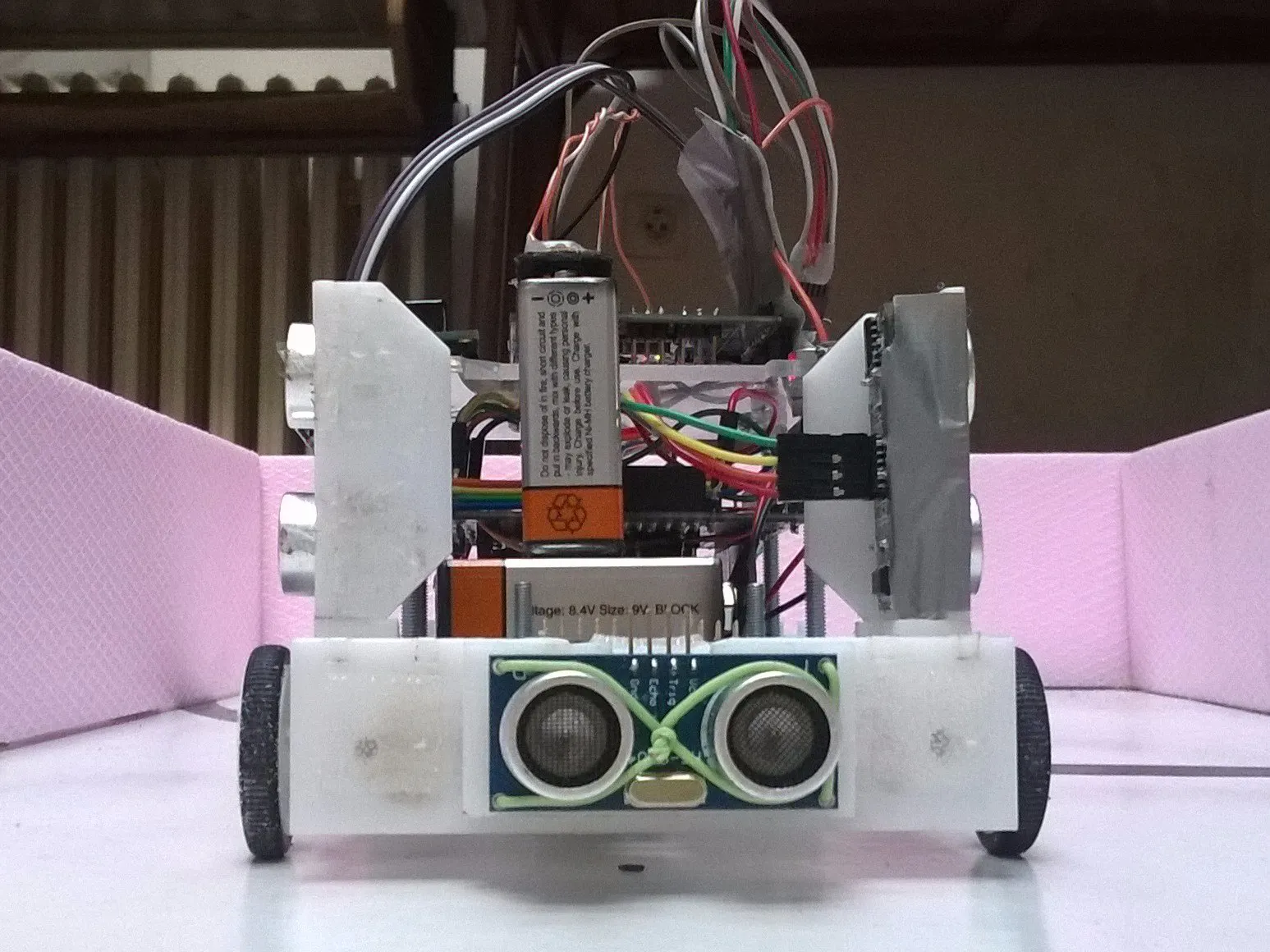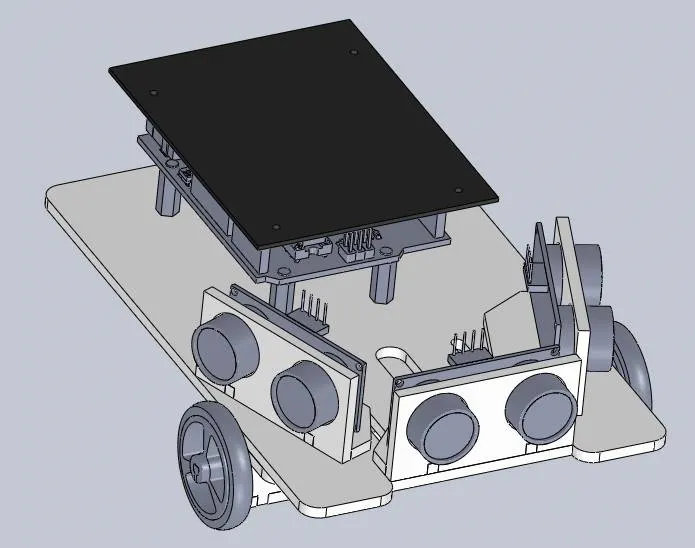Published

# Arduino robot PoliArdo - Maze solver

This robot took part in a competition and solved the maze.## Things used in this project

### Hardware componentsArduino UNO & Genuino UNO
×2
 ultrasonic sensor
×2
 zumo arduino
×1
 color sensor
×1
 DC motor (generic)
×2

### Software apps and online servicesArduino IDE

 laser

## Schematics

### version robot 1## Code

### Code arduino robot

Arduino
arduino board uno its shield arduino zumo control ultrasonic sensors and motors.
```#include <ZumoMotors.h>
#include <ZumoBuzzer.h>
#include <Pushbutton.h>

ZumoBuzzer buzzer;
ZumoMotors motor;
Pushbutton button(ZUMO_BUTTON);
int  f=83.5/80;
int thigD=A2;
int ehcoD=A3;
int thigL=A4;
int ehcoL=A5;
long distanca,trajanje;
int signal=2;

void setup(){
Serial.begin(9600);
pinMode(thigL,OUTPUT);
pinMode(ehcoL,INPUT);
pinMode(thigD,OUTPUT);
pinMode(ehcoD,INPUT);
pinMode(signal, INPUT);
button.waitForButton();
buzzer.play("L16 cdegreg4");
while(buzzer.isPlaying());

DesniZid();
okretDesno();

distancaL=izracunaj(thigL,ehcoL);
LeviZid();
okretLevo();

DesniZid();
okretDesno();

distancaL=izracunaj(thigL,ehcoL);
Prati_Levi_zid();

motorSTOP();
}
void loop(){
}
void motorSTOP(){
motor.setSpeeds(0,0);
delay(500);
}
int izracunaj(int thig,int ehco){

digitalWrite(thig,LOW);
delayMicroseconds(2);
digitalWrite(thig,HIGH);
delayMicroseconds(10);
digitalWrite(thig,LOW);
trajanje=pulseIn(ehco,HIGH);
distanca=(trajanje/2)/29;
return (distanca);
}
void DesniZid(){

motor.setSpeeds(83.5,80); }
motor.setSpeeds(165,55);
delay(15); }
motor.setSpeeds(55,100);
delay(10); }
motor.setSpeeds(100,65);
delay(10); }
}

}
void LeviZid(){
while(distancaL < 27){
distancaL=izracunaj(thigL,ehcoL);

if(distancaL <=11 && distancaL >=9){
motor.setSpeeds(83.5,80);
delay(10); }
else if (distancaL >=15){
motor.setSpeeds(55,165);
delay(15); }
else if(distancaL <9){
motor.setSpeeds(100,55);
delay(10); }
else if(distancaL >11 && distancaL < 15){
motor.setSpeeds(65,100);
delay(10); }
}

}
void Prati_Levi_zid(){
while(1){
distancaL=izracunaj(thigL,ehcoL);
if(distancaL <=11 && distancaL >=9){
motor.setSpeeds(83.5,80);
delay(10); }
else if (distancaL >=15){
motor.setSpeeds(75,165);
delay(15); }
else if(distancaL <9){
motor.setSpeeds(100,72);
delay(10); }
else if(distancaL >11 && distancaL < 15){
motor.setSpeeds(80,100);
delay(10); }
}
}
void okretDesno(){
motorSTOP();
motor.setSpeeds(90*f,90);
delay(1900);
motorSTOP();
motor.setSpeeds(100,-100);
delay(1000);
motorSTOP();
motor.setSpeeds(90*f,90);
delay(4000);
motorSTOP();
motor.setSpeeds(100,-100);
delay(1000);
motorSTOP();

}
void okretLevo(){
motorSTOP();
motor.setSpeeds(90*f,90);
delay(1700);
motorSTOP();
motor.setSpeeds(-100,100);
delay(1000);
motorSTOP();
motor.setSpeeds(90*f,90);
delay(4000);
motorSTOP();
motor.setSpeeds(-100,100);
delay(1000);
motorSTOP();

}
```

### Code arduino robot

Arduino
for arduino board with speaker and color sensor
```#include <Wire.h>
#include <LiquidCrystal_I2C.h>
LiquidCrystal_I2C lcd(0x27, 2, 1, 0, 4, 5, 6, 7, 3, POSITIVE);
#define NOTE_CS8 4435
#define NOTE_B6  1976
int melody[] = {NOTE_CS8,NOTE_B6};
int noteDurations[] = {8,8};
const int s0 = A3;
const int s1 = A4;
const int s2 =A1;
const int s3 = A2;
const int out = A0;
int signal=7;
int ind=0;
int red = 0;
int green = 0;
int blue = 0;
int brojac=0;

void setup(){
Serial.begin(9600);

pinMode(s0, OUTPUT);
pinMode(s1, OUTPUT);
pinMode(s2, OUTPUT);
pinMode(s3, OUTPUT);
pinMode(out, INPUT);
digitalWrite(s0, HIGH);
digitalWrite(s1, HIGH);
pinMode(signal, OUTPUT);
lcd.begin(16,2);
lcd.backlight();
lcd.clear();
lcd.setCursor(0,0);
lcd.print("ARDUINO TEAM");

}
void loop(){
if(ind==0){
color();

delay(200);

if (red < blue && red < green && red < 20)
{
if (!(red <=10 && green <=10 && blue <=10)){
delay(70);
brojac++;
lcd.setCursor(5,1);
lcd.print(brojac);
for (int thisNote = 0; thisNote < 1; thisNote++) {
int noteDuration = 1000/noteDurations[thisNote];
tone(8, melody[thisNote],noteDuration);
int pauseBetweenNotes = noteDuration * 1.30;
delay(pauseBetweenNotes);
noTone(8);
}
}
}

if ((red > 40 && red <80) && (green >20 && green <45) && (blue >0 && blue <20))
{
if (!(red <=10 && green <=10 && blue <=10)){
for (int thisNote = 0; thisNote < 2; thisNote++) {
int noteDuration = 1000/noteDurations[thisNote];
tone(8, melody[thisNote],noteDuration);
int pauseBetweenNotes = noteDuration * 1.30;
delay(pauseBetweenNotes);
noTone(8);
ind++;
}
digitalWrite(signal,HIGH);
}
else digitalWrite(signal,LOW);
}
}
}

void color()
{
digitalWrite(s2, LOW);
digitalWrite(s3, LOW);
red = pulseIn(out, digitalRead(out) == HIGH ? LOW : HIGH);
digitalWrite(s3, HIGH);
blue = pulseIn(out, digitalRead(out) == HIGH ? LOW : HIGH);
digitalWrite(s2, HIGH);
green = pulseIn(out, digitalRead(out) == HIGH ? LOW : HIGH);
}
```

## Credits

### MakerRobotics

6 projects • 117 followers